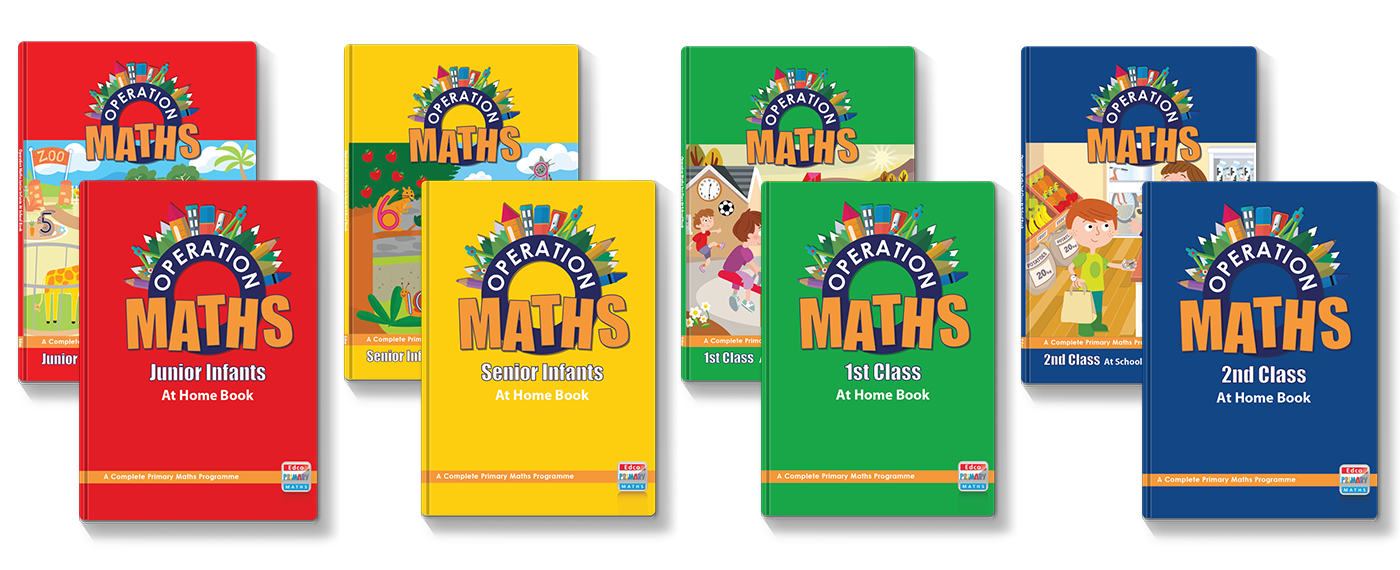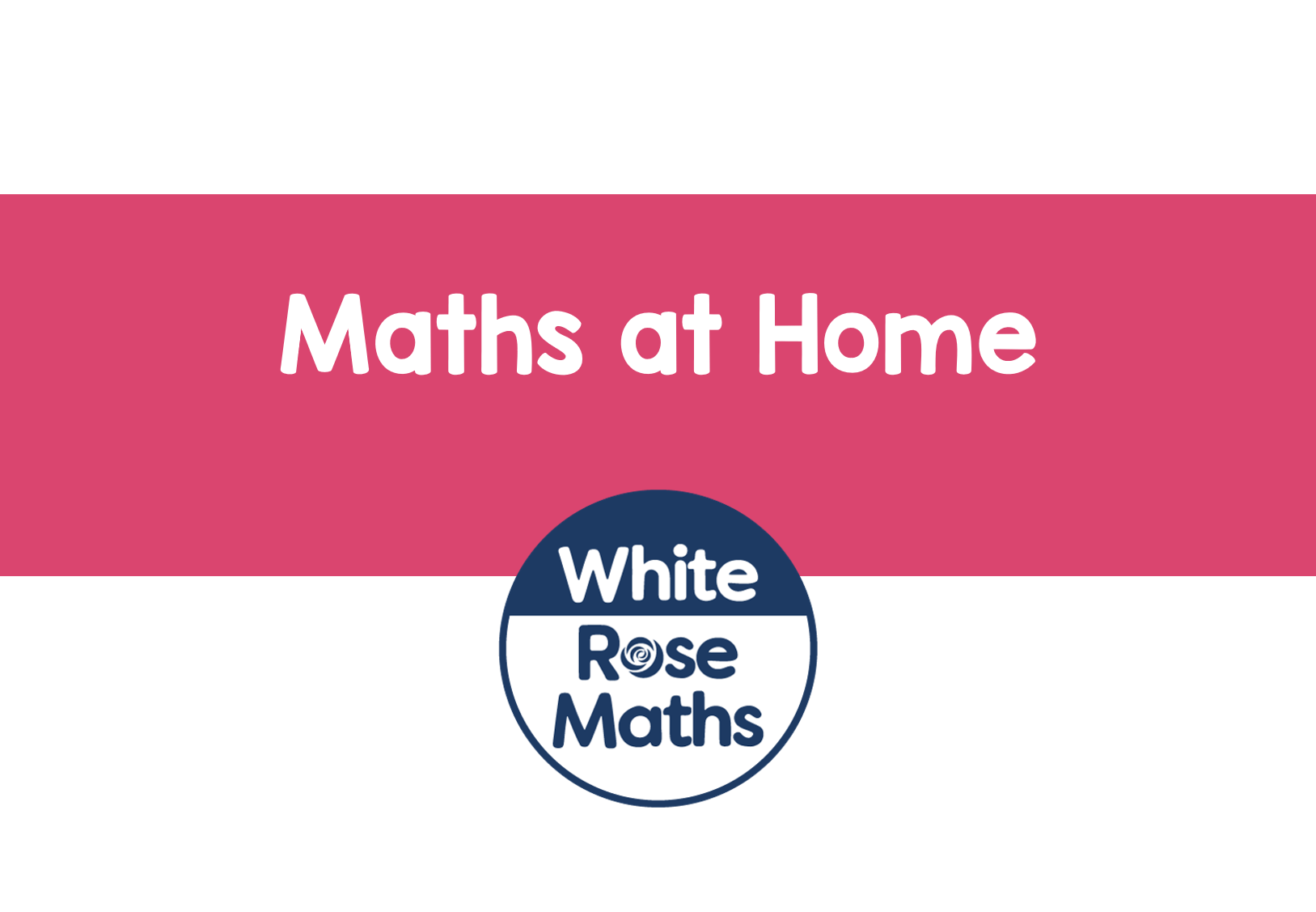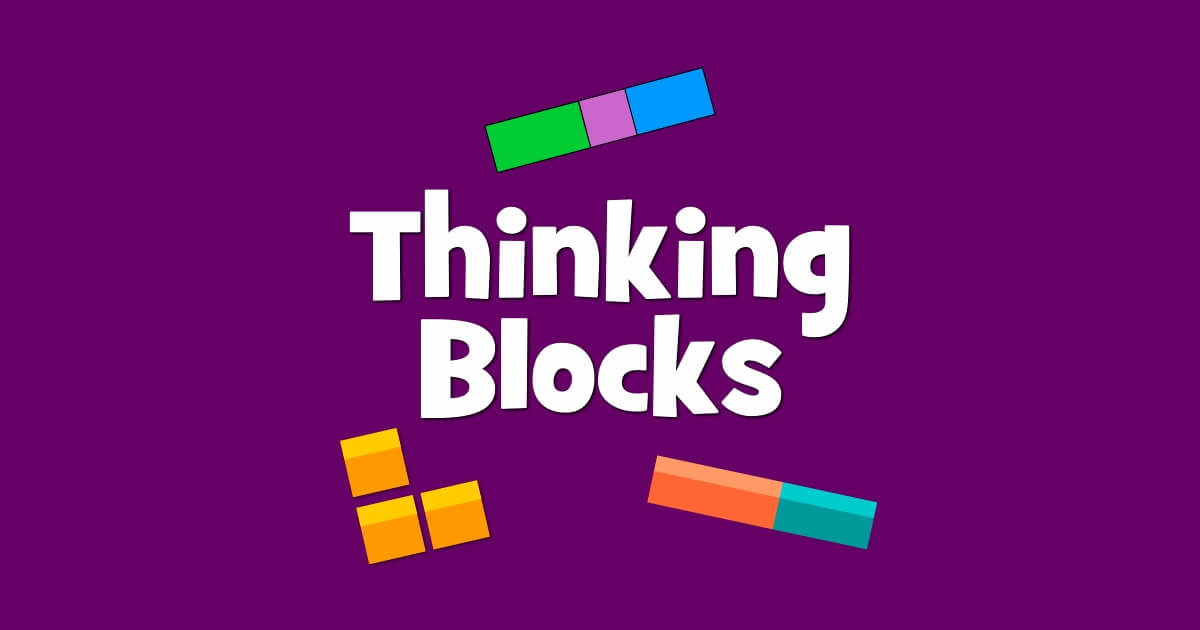# Dear Family, your Operation Maths guide to Multiplication and Division

## Dear Family, your Operation Maths guide to Multiplication and Division

Category : Uncategorized

Dear Family, given below is a brief guide to understanding the topic of multiplication and division, as well as some practical suggestions as to how you might support your children’s understanding at home. Also below, are a series of links to digital resources that will help both the children, and you, learn more about place value. The digital resources are organised according to approximate class level:You can also find class specific tips in the Operation Maths Dear Family letters for third to sixth class.

#### Understanding Multiplication and Division

Like addition and subtraction, multiplication and division is not just about multiplying and dividing to get a correct answer (although accuracy is always to be valued in maths); the focus is very much on understanding and visualising what is happening to the amounts/numbers, how they are changing, why they are changing and how the same calculation can be done in many different ways. This is often referred to as developing a child’s number sense.

In Operation Maths, the children are always encouraged to use materials and pictures, and to explore different strategies (ways) to get their answers. They are also encouraged to compare their strategies with the strategies of others, and to explain or justify why one way might be more efficient (faster and/or simpler) than another way.

In particular, the children are encouraged to refer to some key, benchmark facts, that, in turn, can be used to work out other facts. These include doubling and halving (which can be used to multiply and divide by 2, 4 and 8) and multiplying and dividing by 10, which in turn can be used to multiply by 5 (half 10 times the number) and multiplying by 9 (one group/set less than 10 times).

These benchmark facts (or friendly facts), combined with other strategies, ensures that the child develops both understanding of, and fluency with, the basic multiplication and division facts, which in turn cam be applied to more complex situations later, such as bigger numbers or fractions and decimals. Pictured below are examples of some useful thinking strategies for the basic multiplication and division facts.

While multiplication and division are separate operations, they are also very closely related, being the inverse (or opposite) of each other e.g. 3 x 10 = 30, 30 ÷ 10 = 3, 30 ÷ 3 = 10. This is another strategy that the children can use i.e. think multiplication to do division, or vice versa. Beginning in third and fourth classes, the children will explore multiplication and division as being the inverse of each other, which will progress towards using the inverse (opposite) operation to check their calculations.

Therefore, nowadays, there is less emphasis on just learning off “tables”, (i.e. memorising answers) and more emphasis on thinking strategies to use what they know, to solve what they do not know.

#### Practical Suggestions for Supporting Children

• Invite your child to help out with any opportunity to do multiplication and/or division at home, or when out and about, e.g. how much for 5 packets of the same sweets? If buying a multipack of anything, how much roughly for each item? etc.
• Encourage your child to use strategies when multiplying and dividing and/or to explain their strategies. Ask your child to explain how they know, or worked out the answer.
• “I don’t believe you…prove it!” When your child arrives at an answer, ask him/her to show you how they got an answer, to prove that that is the answer, and encourage them to think of other ways to arrive at the same answer. Are there ways that might be more efficient (better) than others?
• Encourage your child to estimate. Estimation is a very important skill; this involves quickly getting a rough or ‘ballpark’ idea of the answer, e.g. 3 boxes of sweets at €4.79 each is just under €15 because €4.79 is almost €5. Encourage your child to quickly estimate answers e.g. How much, roughly, is that going to cost? Do we have enough money?

#### Digital Resources for Third and Fourth ClassesHappy Numbers Second Grade: Third class pupils could start, and work through Module 6, Foundations of Multiplication and Division, and then progress to Third Grade, Modules 1 and 3. Fourth class pupils could start with Third Grade or go straight to Fourth Grade and work through the activities in Module 2.White Rose Multiplication and Division: a series of lessons on multiplication and division. These lessons could be followed up with other multiplication and division lessons in year 3 and year 4.Khan Academy Third Grade: Third class pupils should start with Intro to Multiplication and then progress through 1-digit Multiplication, Intro to Division and  More with Multiplication and Division.  Watch the videos and then answer the practice questions. Fourth class pupils could work through the Multiplication and Division activities in Fourth Grade. You can also register for a free Khan Academy account to record your progress and explore other topics/grades.The Array: An introduction to rectangular arrays (rows and columns) and how they can be used to understand multiplication.Arithmetic Arrays: Solve multiplication and division questions using the help of rectangular arrays. Three levels in each game.Bunny Times: A game that gradually teaches and tests multiplication in a visual and conceptual way.Partial Product Finder: This is not a game, but it is a tool, that can be used to show an array for multiplication calculations involving 1 or 2 digitsThinking Blocks for Multiplication and Division: an ideal way to start using bar models, or further practice using bar models, to model word problems and computation.Hit the Button: a great site for practicing quick-fire Times Tables and Division Facts. For a slower-paced version of this try the Mental Maths Train, a multiple choice game.That Quiz – Arithmetic: Use this quiz to practice different types of multiplication and division calculations. You can use the options on the left hand side to make the types of calculations easier or more difficult.Multiplication.com: A site dedicated to multiplication games, but also includes division games and games for addition and subtraction.Estimating answers to multiplication and division calculations to win chances to take penalties in a shoot out.I Know it! – Multiplication and Division (Grade three): Scroll down to the multiplication and/or division sections to do any of the activities. If too simple, go to the multiplication and/or division sections in Grade FourSplash Learn – Multiplication & Division Games: An assortment of games organised according to US grade levels; start with the games for third grade level. If too simple, go to the Grade Four games.Multiplication practice games and Division practice games from ixl.com. You can do a number of free quizzes each day without having a subscription. (Please note that the class levels given do not always align accurately with the content of the Irish Primary Curriculum)

#### Digital Resources for Fifth and Sixth ClassesHappy Numbers Fourth Grade: Pupils could start, and work through Module 2, and then progress to Fifth Grade, Module 1 and 2, which includes multiplication and division with decimal numbersWhite Rose Multiplication and Division: a series of lessons on multiplication and division. These lessons could be followed up with other multiplication and division lessons in year 5 and/or year 6.Khan Academy Fourth Grade: Fifth class pupils could try any of the quizzes on multiplication or division to check what they know already, and either re-do some of the units or move on to Fifth Grade. Similarly, sixth class pupils could try the quizzes in Fifth Grade and then re-do or move on to other/new topics as appropriate. You can also register for a free Khan Academy account to record your progress and explore other topics/grades.Arithmetic Arrays: Solve multiplication and division questions using the help of rectangular arrays. Three levels in each game.Partial Product Finder: This is not a game, but it is a tool, that can be used to show an array for multiplication calculations involving 1 or 2 digitsThinking Blocks for Multiplication and Division: an ideal way to start using bar models, or further practice using bar models, to model word problems and computation.Hit the Button: a great site for practicing quick-fire Times Tables and Division Facts.That Quiz – Arithmetic: Use this quiz to practice different types of multiplication and division calculations. You can use the options on the left hand side to make the types of calculations easier or more difficult.Multiplication.com: A site dedicated to multiplication games, but also includes division games and games for addition and subtraction.Estimating answers to multiplication and division calculations to win chances to take penalties in a shoot out.I Know it! – Multiplication and Division (Fifth Grade): Scroll down to the multiplication and/or division sections to do any of the activities. If these are too difficult, go to the multiplication and/or division sections in Grade FourSplash Learn – Multiplication & Division Games: (Fifth Grade) An assortment of games organised according to US grade levels. If these are too difficult, go to the Grade Four games.Multiplication practice games and Division practice games from ixl.com. You can do a number of free quizzes each day without having a subscription. (Please note that the class levels given do not always align accurately with the content of the Irish Primary Curriculum)